# 高频电磁场的多尺度模拟导论

2017年 1月 11日

### 应用范围：天线和无线通信

1. 天线将本地信号转换为自由空间辐射。
2. 辐射向远离天线的方向传播，经过一段相对较长的距离。
3. 另一个天线检测到辐射，并将其转换成接收信号。### 弗里斯传输方程

P_r = p(1-|\Gamma_t|^2)(1-|\Gamma_r|^2)G_t\left(\theta_t,\phi_t\right)G_r\left(\theta_r,\phi_r\right)\left(\frac{\lambda}{4\pi r}\right)^2P_t

• Pt 指发射天线提供给传输线的功率
• Pr 指接收天线从传输线接收的功率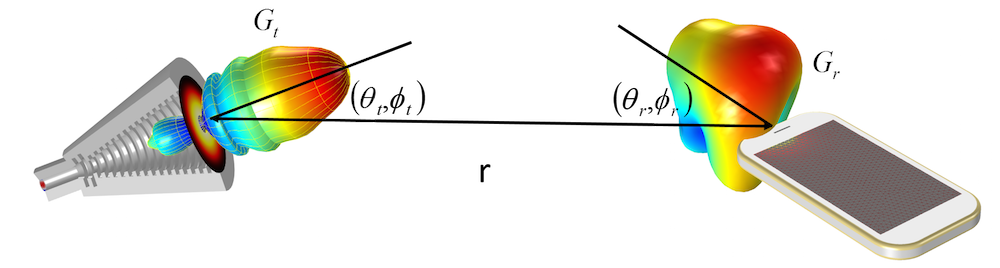### 球面坐标

\begin{align}
r& = sqrt(x^2 + y^2 + z^2)\\
\theta& = acos(z/r)\\
\phi& = atan2(y,x)
\end{align}

\begin{align}
\hat{\theta_x}& = cos(\theta)cos(\phi)\\
\hat{\theta_y}& = cos(\theta)sin(\phi)\\
\hat{\theta_z}& = -sin(\theta)
\end{align}

\hat{r}\hat{\phi} 的笛卡尔分量也能实现类似的任务，但 \hat{\theta} 对于我们来说是最重要的。在介绍射线光学的后续博客中，我们将探讨这一问题。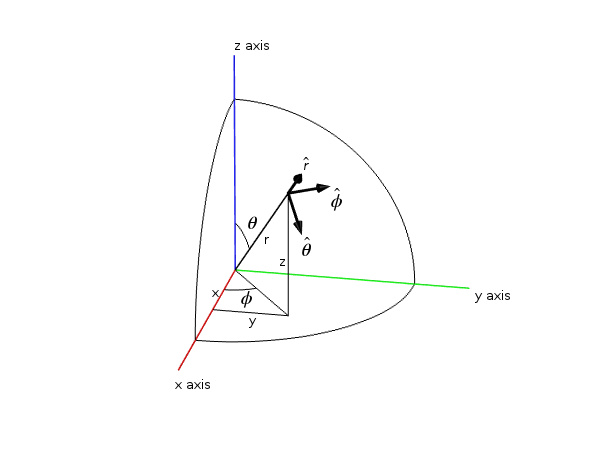### 增益和方向性

1. “增益不包括由阻抗和极化失配所引起的损耗”。
2. “各向同性辐射功率的辐射强度等于天线接收的功率除以 4π”。
3. “如果天线没有耗散损耗，那么在任意给定方向上其增益等于其方向性”。

### 增益、实际增益和阻抗失配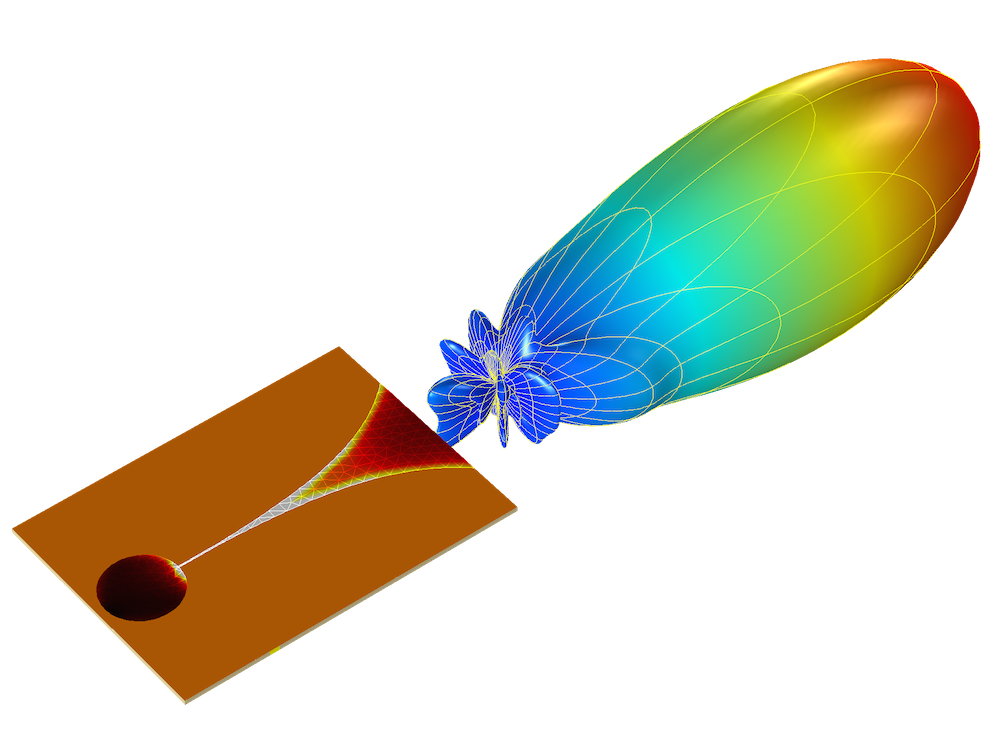Vivaldi 天线的实际增益和电场，使用了 COMSOL Multiphysics 和“RF 模块”进行模拟。您可以在“案例下载”中获取 Vivaldi天线的教学模型

### 发射器示例：完美电偶极子

\overrightarrow{E} =\frac{1}{4\pi\epsilon_0}\{k^2(\hat{r}\times\vec{p})\times\hat{r}\frac{e^{-jkr}}{r}+[3\hat{r}(\hat{r}\cdot\vec{p})-\vec{p}](\frac{1}{r^3}+\frac{jk}{r^2})e^{-jkr}\}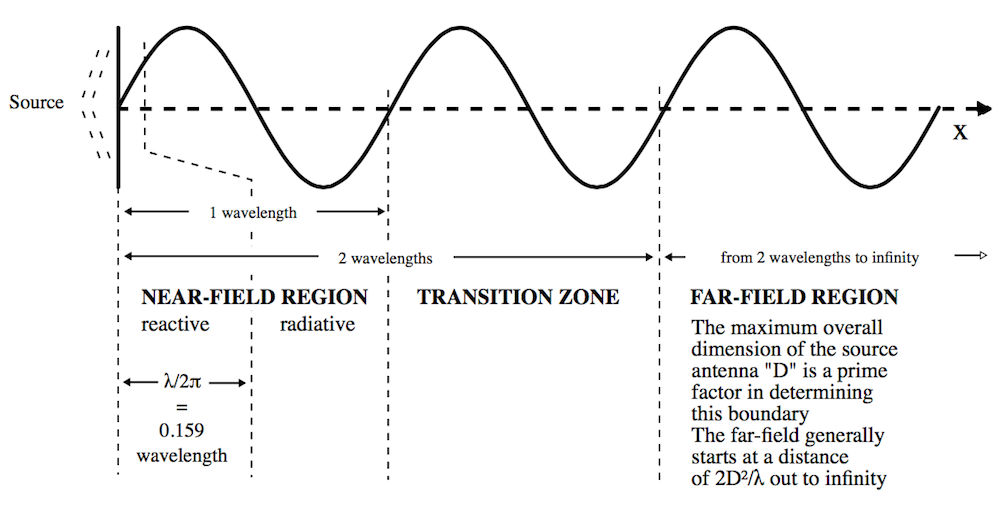\begin{align}
\overrightarrow{E}& = \overrightarrow{E}_{FF} + \overrightarrow{E}_{NF}\\
\overrightarrow{E}_{FF}& = \frac{1}{4\pi\epsilon_0}k^2(\hat{r}\times\vec{p})\times\hat{r}\frac{e^{-jkr}}{r}\\
\overrightarrow{E}_{NF}& = \frac{1}{4\pi\epsilon_0}[3\hat{r}(\hat{r}\cdot\vec{p})-\vec{p}](\frac{1}{r^3}+\frac{jk}{r^2})e^{-jkr}
\end{align}

### 接收器示例：半波长偶极子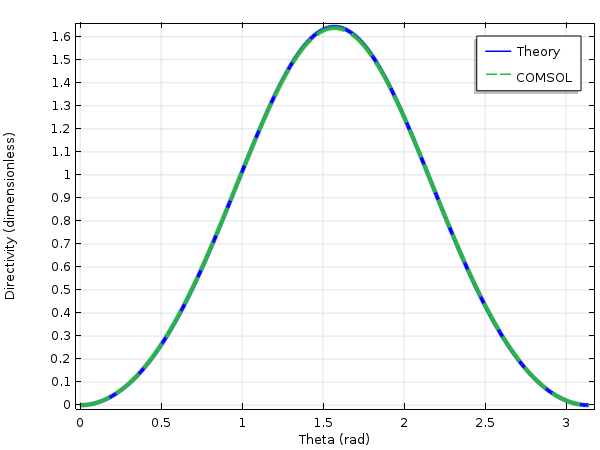### 计算接收功率

P_r = p(1-| \Gamma_r|^2) G_t \left(\theta_t,\phi_t\right) G_r \left(\theta_r,\phi_r\right) \left(\frac{\lambda}{4\pi r}\right)^2 P_{rad}

### 后续博客……

• 在第 2 部分中，我们将使用电磁波，频域 接口模拟电点偶极子的发射过程，并讨论用于计算源的远场辐射的远场域 节点，演示如何将电磁波，频域 接口耦合到电磁波，光束包络 接口，借此模拟中间区的场。
• 在第 3 部分中，我们将模拟点偶极与相距任意距离的半波长偶极天线之间的辐射。为了验证模型，我们将计算半波长偶极天线接收的功率，并使用弗里斯传输方程验证结果。
• 在第 4 部分中，我们将使用几何光学 接口将发射源——点电偶极子耦合到射线光学仿真中。
• 在第 5 部分中，我们将使用几何光学 接口耦合两个天线，并再次验证结果，讨论这种更通用的方法如何用于不均匀的媒介和多路传输。

#### 评论 (1)

##### 留言